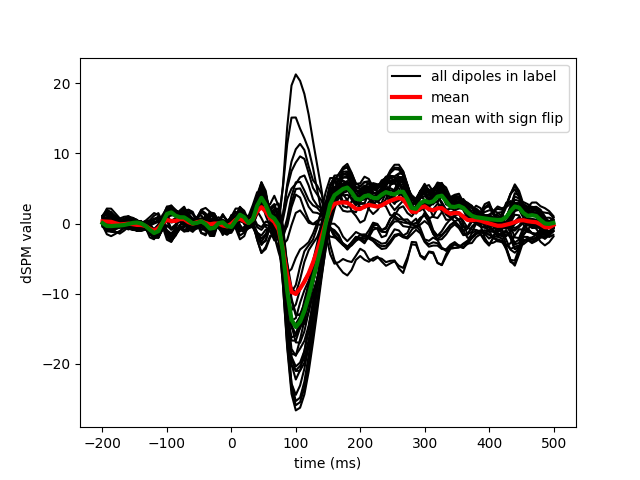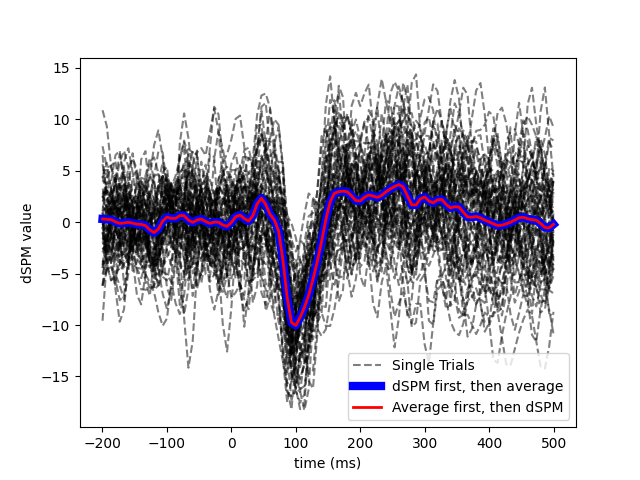# Compute MNE-dSPM inverse solution on single epochs#

Compute dSPM inverse solution on single trial epochs restricted to a brain label.

```# Author: Alexandre Gramfort <alexandre.gramfort@inria.fr>
#
```
```import numpy as np
import matplotlib.pyplot as plt

import mne
from mne.datasets import sample
from mne.minimum_norm import apply_inverse

print(__doc__)

data_path = sample.data_path()
meg_path = data_path / 'MEG' / 'sample'
fname_inv = meg_path / 'sample_audvis-meg-oct-6-meg-inv.fif'
fname_raw = meg_path / 'sample_audvis_filt-0-40_raw.fif'
fname_event = meg_path / 'sample_audvis_filt-0-40_raw-eve.fif'
label_name = 'Aud-lh'
fname_label = meg_path / 'labels' / f'{label_name}.label'

event_id, tmin, tmax = 1, -0.2, 0.5

# Using the same inverse operator when inspecting single trials Vs. evoked
snr = 3.0  # Standard assumption for average data but using it for single trial
lambda2 = 1.0 / snr ** 2

method = "dSPM"  # use dSPM method (could also be MNE or sLORETA)

# Set up pick list
include = []

# pick MEG channels
picks = mne.pick_types(raw.info, meg=True, eeg=False, stim=False, eog=True,
epochs = mne.Epochs(raw, events, event_id, tmin, tmax, picks=picks,
eog=150e-6))

# Get evoked data (averaging across trials in sensor space)
evoked = epochs.average()

# Compute inverse solution and stcs for each epoch
# Use the same inverse operator as with evoked data (i.e., set nave)
# If you use a different nave, dSPM just scales by a factor sqrt(nave)
stcs = apply_inverse_epochs(epochs, inverse_operator, lambda2, method, label,
pick_ori="normal", nave=evoked.nave)

# Mean across trials but not across vertices in label
mean_stc = sum(stcs) / len(stcs)

# compute sign flip to avoid signal cancellation when averaging signed values
flip = mne.label_sign_flip(label, inverse_operator['src'])

label_mean = np.mean(mean_stc.data, axis=0)
label_mean_flip = np.mean(flip[:, np.newaxis] * mean_stc.data, axis=0)

# Get inverse solution by inverting evoked data
stc_evoked = apply_inverse(evoked, inverse_operator, lambda2, method,
pick_ori="normal")

# apply_inverse() does whole brain, so sub-select label of interest
stc_evoked_label = stc_evoked.in_label(label)

# Average over label (not caring to align polarities here)
label_mean_evoked = np.mean(stc_evoked_label.data, axis=0)
```
```Reading inverse operator decomposition from /home/circleci/mne_data/MNE-sample-data/MEG/sample/sample_audvis-meg-oct-6-meg-inv.fif...
[done]
[done]
305 x 305 full covariance (kind = 1) found.
Read a total of 4 projection items:
PCA-v1 (1 x 102) active
PCA-v2 (1 x 102) active
PCA-v3 (1 x 102) active
Average EEG reference (1 x 60) active
22494 x 22494 diagonal covariance (kind = 2) found.
22494 x 22494 diagonal covariance (kind = 6) found.
22494 x 22494 diagonal covariance (kind = 5) found.
Did not find the desired covariance matrix (kind = 3)
Computing patch statistics...
[done]
Computing patch statistics...
[done]
Read a total of 4 projection items:
PCA-v1 (1 x 102) active
PCA-v2 (1 x 102) active
PCA-v3 (1 x 102) active
Average EEG reference (1 x 60) active
Source spaces transformed to the inverse solution coordinate frame
Opening raw data file /home/circleci/mne_data/MNE-sample-data/MEG/sample/sample_audvis_filt-0-40_raw.fif...
Read a total of 4 projection items:
PCA-v1 (1 x 102)  idle
PCA-v2 (1 x 102)  idle
PCA-v3 (1 x 102)  idle
Average EEG reference (1 x 60)  idle
Range : 6450 ... 48149 =     42.956 ...   320.665 secs
72 matching events found
Setting baseline interval to [-0.19979521315838786, 0.0] sec
Applying baseline correction (mode: mean)
Created an SSP operator (subspace dimension = 3)
4 projection items activated
Rejecting  epoch based on EOG : ['EOG 061']
Rejecting  epoch based on EOG : ['EOG 061']
Rejecting  epoch based on EOG : ['EOG 061']
Rejecting  epoch based on EOG : ['EOG 061']
Rejecting  epoch based on EOG : ['EOG 061']
Rejecting  epoch based on MAG : ['MEG 1711']
Rejecting  epoch based on EOG : ['EOG 061']
Rejecting  epoch based on EOG : ['EOG 061']
Rejecting  epoch based on EOG : ['EOG 061']
Rejecting  epoch based on EOG : ['EOG 061']
Rejecting  epoch based on EOG : ['EOG 061']
Rejecting  epoch based on EOG : ['EOG 061']
Rejecting  epoch based on EOG : ['EOG 061']
Rejecting  epoch based on EOG : ['EOG 061']
Rejecting  epoch based on EOG : ['EOG 061']
Rejecting  epoch based on EOG : ['EOG 061']
Rejecting  epoch based on EOG : ['EOG 061']
Removing projector <Projection | Average EEG reference, active : True, n_channels : 60>
Preparing the inverse operator for use...
Scaled noise and source covariance from nave = 1 to nave = 55
Created the regularized inverter
Created an SSP operator (subspace dimension = 3)
Created the whitener using a noise covariance matrix with rank 302 (3 small eigenvalues omitted)
Computing noise-normalization factors (dSPM)...
[done]
Picked 305 channels from the data
Computing inverse...
Eigenleads need to be weighted ...
Processing epoch : 1 / 72 (at most)
Processing epoch : 2 / 72 (at most)
Processing epoch : 3 / 72 (at most)
Processing epoch : 4 / 72 (at most)
Processing epoch : 5 / 72 (at most)
Processing epoch : 6 / 72 (at most)
Processing epoch : 7 / 72 (at most)
Processing epoch : 8 / 72 (at most)
Processing epoch : 9 / 72 (at most)
Processing epoch : 10 / 72 (at most)
Rejecting  epoch based on EOG : ['EOG 061']
Processing epoch : 11 / 72 (at most)
Processing epoch : 12 / 72 (at most)
Processing epoch : 13 / 72 (at most)
Rejecting  epoch based on EOG : ['EOG 061']
Rejecting  epoch based on EOG : ['EOG 061']
Processing epoch : 14 / 72 (at most)
Processing epoch : 15 / 72 (at most)
Processing epoch : 16 / 72 (at most)
Processing epoch : 17 / 72 (at most)
Rejecting  epoch based on EOG : ['EOG 061']
Processing epoch : 18 / 72 (at most)
Processing epoch : 19 / 72 (at most)
Processing epoch : 20 / 72 (at most)
Rejecting  epoch based on EOG : ['EOG 061']
Processing epoch : 21 / 72 (at most)
Processing epoch : 22 / 72 (at most)
Processing epoch : 23 / 72 (at most)
Rejecting  epoch based on MAG : ['MEG 1711']
Processing epoch : 24 / 72 (at most)
Processing epoch : 25 / 72 (at most)
Processing epoch : 26 / 72 (at most)
Processing epoch : 27 / 72 (at most)
Processing epoch : 28 / 72 (at most)
Rejecting  epoch based on EOG : ['EOG 061']
Processing epoch : 29 / 72 (at most)
Processing epoch : 30 / 72 (at most)
Processing epoch : 31 / 72 (at most)
Rejecting  epoch based on EOG : ['EOG 061']
Processing epoch : 32 / 72 (at most)
Processing epoch : 33 / 72 (at most)
Rejecting  epoch based on EOG : ['EOG 061']
Processing epoch : 34 / 72 (at most)
Processing epoch : 35 / 72 (at most)
Rejecting  epoch based on EOG : ['EOG 061']
Rejecting  epoch based on EOG : ['EOG 061']
Processing epoch : 36 / 72 (at most)
Rejecting  epoch based on EOG : ['EOG 061']
Rejecting  epoch based on EOG : ['EOG 061']
Processing epoch : 37 / 72 (at most)
Processing epoch : 38 / 72 (at most)
Processing epoch : 39 / 72 (at most)
Processing epoch : 40 / 72 (at most)
Processing epoch : 41 / 72 (at most)
Processing epoch : 42 / 72 (at most)
Processing epoch : 43 / 72 (at most)
Processing epoch : 44 / 72 (at most)
Rejecting  epoch based on EOG : ['EOG 061']
Processing epoch : 45 / 72 (at most)
Rejecting  epoch based on EOG : ['EOG 061']
Processing epoch : 46 / 72 (at most)
Processing epoch : 47 / 72 (at most)
Processing epoch : 48 / 72 (at most)
Rejecting  epoch based on EOG : ['EOG 061']
Rejecting  epoch based on EOG : ['EOG 061']
Processing epoch : 49 / 72 (at most)
Processing epoch : 50 / 72 (at most)
Processing epoch : 51 / 72 (at most)
Processing epoch : 52 / 72 (at most)
Processing epoch : 53 / 72 (at most)
Processing epoch : 54 / 72 (at most)
Processing epoch : 55 / 72 (at most)
[done]
Preparing the inverse operator for use...
Scaled noise and source covariance from nave = 1 to nave = 55
Created the regularized inverter
Created an SSP operator (subspace dimension = 3)
Created the whitener using a noise covariance matrix with rank 302 (3 small eigenvalues omitted)
Computing noise-normalization factors (dSPM)...
[done]
Applying inverse operator to "1"...
Picked 305 channels from the data
Computing inverse...
Eigenleads need to be weighted ...
Computing residual...
Explained  59.3% variance
dSPM...
[done]
```

View activation time-series to illustrate the benefit of aligning/flipping

```times = 1e3 * stcs.times  # times in ms

plt.figure()
h0 = plt.plot(times, mean_stc.data.T, 'k')
h1, = plt.plot(times, label_mean, 'r', linewidth=3)
h2, = plt.plot(times, label_mean_flip, 'g', linewidth=3)
plt.legend((h0, h1, h2), ('all dipoles in label', 'mean',
'mean with sign flip'))
plt.xlabel('time (ms)')
plt.ylabel('dSPM value')
plt.show()
```Viewing single trial dSPM and average dSPM for unflipped pooling over label Compare to (1) Inverse (dSPM) then average, (2) Evoked then dSPM

```# Single trial
plt.figure()
for k, stc_trial in enumerate(stcs):
plt.plot(times, np.mean(stc_trial.data, axis=0).T, 'k--',
label='Single Trials' if k == 0 else '_nolegend_',
alpha=0.5)

# Single trial inverse then average.. making linewidth large to not be masked
plt.plot(times, label_mean, 'b', linewidth=6,
label='dSPM first, then average')

# Evoked and then inverse
plt.plot(times, label_mean_evoked, 'r', linewidth=2,
label='Average first, then dSPM')

plt.xlabel('time (ms)')
plt.ylabel('dSPM value')
plt.legend()
plt.show()
```Total running time of the script: ( 0 minutes 6.172 seconds)

Estimated memory usage: 10 MB

Gallery generated by Sphinx-Gallery